Geoboard Magic

I started with making a shape with 4 touching nails on the geoboard, 1 unit of area.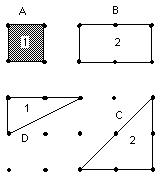I had to figure out a rule for finding the area (y) inside the shape,  with different numbers of nails  touching the rubberband (x) and the number of nails inside the figure (i).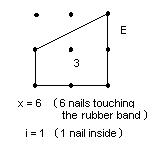I wrote down how many units of area would be inside a figure depending on x and made a table, first for no nails inside: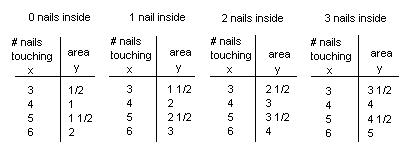I found patterns in the table, then I wrote the rule as

x - (x+2)/2 = y

Both Patricia and Paul graphed the pairs of numbers (x, y) from their tables, for each i.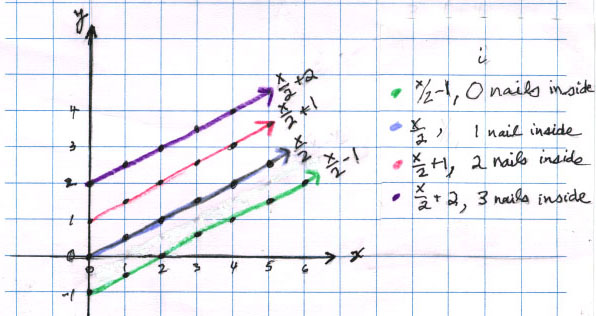They then were able to pick off the slope (1/2 for each one) and the intercept, easily, and write the rules more simply as

x/2 + -1 = y ,  when i=0

x/2 +  0 = y ,   when i=1

x/2 +  1 = y ,   when i=2,   and so on.

The rule with i was simple to see, because the adding number was 1 less than i. They wrote the 3-variable rule as

x/2 + i - 1 = y  (also known as Pick's theorem)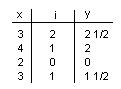They checked this rule with the data they had and it worked!

Don helped Paul, then Maggie, make the 3-D graph of the area y in terms of x and i using the table above. The 3-D graphing board they used was a peg-board and dowel rods, marked off in units. The points were made with rubber bands. This 3-D graphing board was invented by Theo when he was in 5th grade- the same person who years later helped Don with his book "Changing Shapes With Matrices"! It looked like this: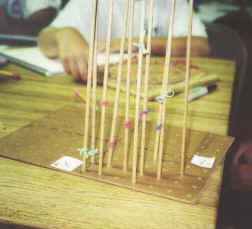3D graph of  the area y (vertical axis) in terms of x, the number of nails touching the rubber band (coming towards you)  and i the number of nails inside (going to the right). Check out DPGraph.com to graph in 3D on line- great!

==========================================

To other discoveries